# JP5591194B2 - Motor drive device - Google Patents

## Info

Publication number
JP5591194B2
JP5591194B2 JP2011188530A JP2011188530A JP5591194B2 JP 5591194 B2 JP5591194 B2 JP 5591194B2 JP 2011188530 A JP2011188530 A JP 2011188530A JP 2011188530 A JP2011188530 A JP 2011188530A JP 5591194 B2 JP5591194 B2 JP 5591194B2
Authority
JP
Japan
Prior art keywords
current
current detection
detection circuit
current value
shunt resistor
Prior art date
Legal status (The legal status is an assumption and is not a legal conclusion. Google has not performed a legal analysis and makes no representation as to the accuracy of the status listed.)
Active
Application number
JP2011188530A
Other languages
Japanese (ja)
Other versions
JP2012075307A (en
Inventor

Original Assignee
オムロンオートモーティブエレクトロニクス株式会社
Priority date (The priority date is an assumption and is not a legal conclusion. Google has not performed a legal analysis and makes no representation as to the accuracy of the date listed.)
Filing date
Publication date
Priority to JP2010196458 priority Critical
Priority to JP2010196458 priority
Application filed by オムロンオートモーティブエレクトロニクス株式会社 filed Critical オムロンオートモーティブエレクトロニクス株式会社
Priority to JP2011188530A priority patent/JP5591194B2/en
Publication of JP2012075307A publication Critical patent/JP2012075307A/en
Application granted granted Critical
Publication of JP5591194B2 publication Critical patent/JP5591194B2/en
Application status is Active legal-status Critical
Anticipated expiration legal-status Critical

## Images

•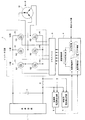•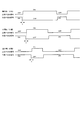•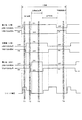•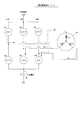••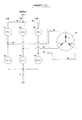••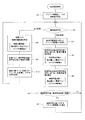••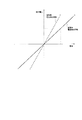•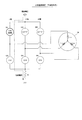•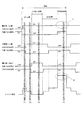••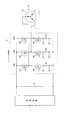## Description

The present invention relates to a motor drive device using a PWM (Pulse Width Modulation) control system, and more particularly to a technique for compensating for a drift current flowing in a shunt resistor for current detection.

In an electric power steering device for a vehicle, an electric motor such as a brushless motor is provided in order to give a steering assist force to the steering mechanism in accordance with the steering torque of the steering wheel. As a device for driving this motor, a motor drive device using a PWM control system is known.

In general, a PWM control type motor drive device includes an inverter circuit and a control unit that controls the inverter circuit. The inverter circuit is provided with a plurality of sets (three sets in the case of a three-phase motor) of a pair of upper and lower arms each having a switching element on the upper arm and the lower arm. Further, the control unit calculates a target value of the current to be passed through the motor according to the steering torque detected by the torque sensor, and determines a predetermined value based on a deviation between the target value and the value of the current actually flowing through the motor. A PWM signal having a duty is generated. The PWM signal controls the ON / OFF operation of each switching element of the inverter circuit, and the motor is driven by the voltage output from the inverter circuit in accordance with this.

In the motor driving device as described above, the current flowing through the motor is detected using a current detection resistor called a shunt resistor. By the way, in a period in which all the upper switching elements in the inverter circuit are OFF, or in a period in which all the lower switching elements are OFF, the inverter circuit is in a regenerative state and theoretically no current flows through the shunt resistor. However, in reality, current may flow through the shunt resistor even during the above period due to the influence of temperature, noise, and the like. This current is called drift current. Although the drift current is very small, the current flows in a period in which the detected current value should be essentially zero, which affects the steering assist force and gives the driver a sense of incongruity in terms of steering feeling.

Therefore, it is necessary to compensate the detected current value based on the drift current so that the drift current does not affect the steering assist force. The following Patent Document 1 describes a technique relating to drift current compensation. In this document, the current flowing through the motor is monitored by a shunt resistor, the output of the shunt resistor is measured at the timing when the instantaneous current flowing through this resistor becomes substantially zero, and the current between the actual measured output value and the ideal output value is measured. A signal for compensating for the drift (offset) of the signal is generated.

JP 2001-95279 A

In the apparatus of Patent Document 1, when only one circuit for detecting the current flowing through the shunt resistor is provided, if the detection circuit fails, current detection cannot be performed and motor control becomes impossible. Therefore, by providing two current detection circuits and duplicating them, even if one current detection circuit fails, the other current detection circuit can detect the motor current and improve the reliability. .

When the current detection circuit is duplicated and the drift current flows through the shunt resistor, the current detection value of both current detection circuits should be the same value. Due to the variation, a deviation occurs in the current detection value. In addition, since the drift current flowing through the shunt resistor fluctuates depending on the temperature, if the temperature rises with time, the deviation of the current detection value increases between the respective current detection circuits, resulting in trouble in motor control.

An object of the present invention is to provide a motor drive device that can perform appropriate control even when the current detection circuit is duplicated.

The motor driving device according to the present invention includes a plurality of pairs of upper and lower arms each having a switching element on an upper arm and a lower arm, and the motor is driven based on ON / OFF operation of each switching element by a PWM (Pulse Width Modulation) signal. An inverter circuit for driving the motor, a shunt resistor for detecting a current flowing from the motor through the inverter circuit, a first current detection circuit for monitoring for detecting a current flowing in the shunt resistor, and a current flowing in the shunt resistor A control second current detection circuit to detect, and a control means for generating a PWM signal based on the current value obtained from the output of the second current detection circuit and the target value of the current to be passed through the motor.

When the inverter circuit is in a regenerative state, the control means includes a first drift compensation for compensating for a drift current flowing through the shunt resistor with respect to the monitoring current value calculated based on the output of the first current detection circuit. And a second drift compensation amount for compensating for the drift current flowing through the shunt resistor, respectively, with respect to the control current value calculated based on the amount and the output of the second current detection circuit. When the first current detection circuit detects that the drift current has flowed through the shunt resistor, the control unit corrects the first drift compensation amount and uses the corrected first drift compensation amount. A monitoring current value is calculated, and a control current value is calculated using the second drift compensation amount before correction. If the difference between the calculated monitoring current value and the control current value is larger than the threshold value, the second drift compensation amount is corrected so that the control current value becomes zero. Here, zero is a concept including not only numerical zero but also substantial zero including an error range (the same applies hereinafter).

In the present invention, when one of the two current detection circuits is used for monitoring and the other is used for control, the control current value is compared with the monitor current value, and the control current value is deviated from the monitor current value by a predetermined amount. The compensation is performed so that the control current value becomes zero. As a result, even if a drift current flows through the shunt resistor during regeneration, the control current value is compensated to zero, so that the motor behavior is not affected. For this reason, for example, in an electric power steering apparatus, since a drift current does not affect the steering assist force, a stable steering feeling can be obtained. Even if the drift current increases due to a temperature rise, if the deviation between the control current value and the monitoring current value becomes large, compensation for the control current value is executed, and therefore, based on the compensated control current value. Therefore, proper control can be performed. In addition, in the present invention, the control current value is compensated only when the difference between the current values becomes larger than the threshold value, so that the control current value that is the basis for generating the PWM signal fluctuates more than necessary. There is no. For this reason, a more stable steering feeling can be maintained.

According to a preferred embodiment of the present invention, the control means determines whether the current value of the current flowing through the shunt resistor is zero based on the output of the first current detection circuit when the inverter circuit is in the regenerative state. Determine. If the current value is not zero, the drift current flows through the shunt resistor, so the first drift compensation amount is corrected. On the other hand, when the current value is zero, the first drift compensation amount is not corrected because no drift current flows through the shunt resistor.

According to a preferred embodiment of the present invention, the current detection range by the first current detection circuit is wider than the current detection range by the second current detection circuit.

In this way, since the range of the current detected by the first current detection circuit can be widened, the first current detection circuit can be used not only for the monitoring current but also for the shunt resistor when the inverter circuit is in the regenerative state. A large current exceeding a predetermined value can be detected. This makes it possible to determine that at least one of the switching elements has a short circuit failure.

Further, according to a preferred embodiment of the present invention, the shunt resistor is a single resistor, and the voltage generated across the shunt resistor is commonly applied to the first current detection circuit and the second current detection circuit. . Thereby, current detection can be performed using a minimum shunt resistance.

According to the present invention, it is possible to provide a motor drive device capable of performing appropriate control even when the current detection circuit is duplicated.

It is the figure which showed the motor drive device which concerns on embodiment of this invention. It is the figure which showed the PWM signal of each phase. It is a figure explaining the timing of an electric current detection. It is the figure which showed the electric current path | route at the time of normal electric current detection. It is the figure which showed the electric current path | route at the time of normal electric current detection. It is the figure which showed the electric current path at the time of an upper stage regeneration. It is the figure which showed the electric current path | route at the time of lower stage regeneration. It is the flowchart which showed the procedure of drift compensation. It is the flowchart which showed the procedure of drift compensation amount correction | amendment. It is a figure explaining an electric current detection range. It is a figure explaining the short circuit failure at the time of lower stage regeneration. It is the figure which showed the PWM signal and shunt voltage at the time of a short circuit failure. It is a figure explaining the short circuit failure at the time of upper stage regeneration. It is the figure which showed the motor drive device which concerns on other embodiment of this invention.

Hereinafter, embodiments of the present invention will be described with reference to the drawings.

FIG. 1 shows an example of a PWM control type motor drive device using a single shunt resistor. The power supply circuit 1 includes a rectifier circuit, a smoothing circuit, and the like, and a capacitor C is connected to the output end. The inverter circuit 2 is composed of a three-phase bridge in which a pair of upper and lower arms is provided in three sets corresponding to the U phase, V phase, and W phase. The U-phase upper arm A1 has a switching element Q1, and the U-phase lower arm A2 has a switching element Q2. The V-phase upper arm A3 has a switching element Q3, and the V-phase lower arm A4 has a switching element Q4. The W-phase upper arm A5 has a switching element Q5, and the W-phase lower arm A6 has a switching element Q6. These switching elements Q1 to Q6 are made of FET (Field Effect Transistor).

The motor M is a three-phase brushless motor used in, for example, an electric power steering device for a vehicle. A shunt resistor R for detecting a current flowing through the motor M is connected between the power supply circuit 1 and the inverter circuit 2. The shunt resistor R is composed of a single resistor, and the voltage across the shunt resistor R is applied to the monitoring current detection circuit 5 (first current detection circuit) and the control current detection circuit 6 (second current detection circuit). Commonly given. The current detection circuits 5 and 6 amplify the voltage across the shunt resistor R and output the amplified voltage to the arithmetic control unit 4. The arithmetic control unit 4 includes a CPU, a memory, and the like, and constitutes a control means in the present invention. The arithmetic control unit 4 is a target current value (motor M) calculated from a control current value obtained from the output of the control current detection circuit 6 and a detected torque value given from a torque sensor (not shown) for detecting steering torque. The duty of the PWM signal for each phase is calculated on the basis of the deviation from the target value of the current to be passed through. Then, the PWM signal of each phase generated based on this duty and the sawtooth carrier signal is supplied to the driver IC 3. The arithmetic control unit 4 performs drift compensation on the control current value based on the comparison result between the monitoring current value obtained from the output of the monitoring current detection circuit 5 and the control current value (for details, see FIG. Later). The driver IC 3 outputs a PWM signal of each phase for individually turning ON / OFF the switching elements Q1 to Q6 to the gates of the switching elements Q1 to Q6. A three-phase voltage is supplied from the inverter circuit 2 to the motor M by the ON / OFF operation of the switching elements Q1 to Q6 based on the PWM signal, and the motor M rotates.

FIG. 2 shows a PWM signal supplied from the driver IC 3 to the inverter circuit 2. Here, the U phase is the largest phase with the largest duty, the V phase is the intermediate phase with the middle duty, and the W phase is the smallest phase with the smallest duty. The U-phase upper PWM signal is a PWM signal applied to the switching element Q1 of the upper arm A1, and the lower PWM signal is a PWM signal applied to the switching element Q2 of the lower arm A2. The V-phase upper PWM signal is a PWM signal applied to the switching element Q3 of the upper arm A3, and the lower PWM signal is a PWM signal applied to the switching element Q4 of the lower arm A4. The W-phase upper PWM signal is a PWM signal applied to the switching element Q5 of the upper arm A5, and the lower PWM signal is a PWM signal applied to the switching element Q6 of the lower arm A6. In the figure, τ is a dead time for avoiding a short circuit occurring in the inverter circuit 2 when the upper and lower switching elements in each arm are simultaneously turned on.

Next, the timing for detecting the current flowing through the motor M will be described with reference to FIG. As shown in FIG. 3, in one cycle of the PWM signal, there are a power running section and a regeneration section (an upper stage regeneration section and a lower stage regeneration section). In the power running section, except for the dead time section, at least one of the upper arm switching elements (hereinafter referred to as “upper switching elements”) Q1, Q3, and Q5 of the inverter circuit 2 is turned on, and the lower arm switching element ( The motor current flows through the shunt resistor R when at least one of Q2, Q4, and Q6 (hereinafter referred to as “lower switching element”) is turned on. Therefore, the shunt voltage shown in the figure appears at both ends of the shunt resistor R.

On the other hand, since the motor current does not flow through the shunt resistor R in the upper regeneration section where the upper switching elements Q1, Q3, and Q5 are all in the ON state and the lower switching elements Q2, Q4, and Q6 are all in the OFF state, Shunt voltage does not appear at both ends. Even in the lower stage regeneration section where the upper switching elements Q1, Q3, and Q5 are all in the OFF state and the lower switching elements Q2, Q4, and Q6 are all in the ON state, the motor current does not flow through the shunt resistor R. Shunt voltage does not appear at both ends.

T1 to T4 indicate timings for detecting the current flowing through the shunt resistor R (that is, the current of the motor M). Here, the timing is a concept of a section having a time width in which current detection is possible. Timings T1 and T2 are detection timings in the power running section, and these are normal current detection timings when the motor current is flowing through the shunt resistor R. Timings T3 and T4 are detection timings in the upper regeneration zone and the lower regeneration zone, respectively, and these are current detection timings when no current flows in the shunt resistor R in theory.

At timing T1 in FIG. 3, the upper switching elements Q1, Q3, and Q5 are ON, OFF, and OFF, respectively, and the lower switching elements Q2, Q4, and Q6 are respectively OFF, ON, and ON. Current flows along the path indicated by the broken-line arrow. At this time, the current flowing through the shunt resistor R is a U-phase current, and the U-phase current of the motor M is detected at timing T1.

At timing T2 in FIG. 3, the upper switching elements Q1, Q3, and Q5 are ON, ON, and OFF, respectively, and the lower switching elements Q2, Q4, and Q6 are respectively OFF, OFF, and ON. A current flows through a path indicated by a broken-line arrow. At this time, the current flowing through the shunt resistor R is a W-phase current, and the W-phase current of the motor M is detected at timing T2.

The V-phase current is obtained by calculation from the U-phase current and the W-phase current. That is, when the U-phase current value is Iu, the V-phase current value is Iv, and the W-phase current value is Iw, the following relationship is established according to Kirchhoff's law.
Iu + Iv + Iw = 0
Therefore, the V-phase current value Iv is
Iv = − (Iu + Iw)
Can be calculated as

At timing T3 in FIG. 3, the upper switching elements Q1, Q3, and Q5 are all turned on, and the lower switching elements Q2, Q4, and Q6 are all turned off. The inverter circuit 2 receives current along the path indicated by the broken line arrow in FIG. Flowing. The current at this time is a regenerative current due to the discharge of electrical energy accumulated in the windings Lu, Lv, and Lw of the motor M, and is circulated through the upper switching elements Q1, Q3, and Q5. Therefore, theoretically, no current should flow through the shunt resistor R at the timing T3. However, as described above, a drift current may flow through the shunt resistor R due to the influence of temperature, noise, and the like. In this case, since a voltage is generated at both ends of the shunt resistor R, the drift current is detected based on this voltage.

At timing T4 in FIG. 3, the upper switching elements Q1, Q3, and Q5 are all OFF, and the lower switching elements Q2, Q4, and Q6 are all ON. The inverter circuit 2 receives current along the path indicated by the broken line arrow in FIG. Flowing. The current at this time is a regenerative current due to the discharge of electric energy accumulated in the windings Lu, Lv, and Lw of the motor M, and is circulated through the lower switching elements Q2, Q4, and Q6. Therefore, theoretically, no current should flow through the shunt resistor R even at the timing T4, but a drift current may flow through the shunt resistor R for the same reason as described above. Also in this case, since a voltage is generated across the shunt resistor R, the drift current is detected based on this voltage.

Next, drift compensation performed by the arithmetic control unit 4 based on the outputs of the monitoring current detection circuit 5 and the control current detection circuit 6 will be described.

The drift compensation is performed in order to prevent the steering assist force from being influenced by the drift current flowing through the shunt resistor R when the inverter circuit 2 is in the regenerative state, that is, in a period in which no current flows theoretically through the shunt resistor R. This is a detection current value correction process. In executing this drift compensation, in the present embodiment, a first drift compensation amount for performing drift compensation on the monitoring current value calculated based on the output of the monitoring current detection circuit 5, and a control current detection circuit And a second drift compensation amount for performing drift compensation on the control current value calculated based on the output of 6.

In the regeneration section (timing T3, T4 in FIG. 3), the current flowing through the shunt resistor R is monitored by the monitoring current detection circuit 5. When it is detected that the drift current has flowed through the shunt resistor R, first, the first drift compensation amount is corrected by the correction value, and the monitoring current value is calculated using the corrected drift compensation amount. Next, a control current value is calculated using the second drift compensation amount before correction. The monitoring current value at this time is a target value calculated using the compensation amount after correction, and the control current value is a current value calculated using the compensation amount before correction. Therefore, the calculated monitoring current value is compared with the control current value. As a result of the comparison, if the difference between the two (control current value−monitor current value) is larger than the threshold value, it is determined that the control current value has deviated from the monitor current value beyond a certain limit, The second drift compensation amount is corrected. The control current value is compensated to be zero by the corrected second drift compensation amount.

On the other hand, in the power running section (timing T1, T2 in FIG. 3), the output of the monitoring current detection circuit 5 is ignored, and based on the output of the control current detection circuit 6 and the corrected second drift compensation amount. Then, the control current value is calculated, and the duty of the PWM signal of each phase is calculated by the conventional method using the control current value and the target current value.

Next, the drift compensation procedure will be described in more detail with reference to the flowchart of FIG. Each step in FIG. 8 is executed by the CPU constituting the calculation control unit 4. When current detection is started, first, initial values of the drift compensation amounts α and β are set in step S1. Here, α is the first drift compensation amount, and β is the second drift compensation amount. The initial values of α and β are set to zero, for example.

Subsequently, in step S2, it is determined whether the current detection section is a regeneration section or a power running section. This determination is made based on the ON / OFF patterns of the switching elements Q1 to Q6.

If the determination result in step S2 is a power running section, the process proceeds to step S8 to calculate a control current value for each phase for the motor M (three-phase brushless motor). Specifically, first, the control current detection circuit 6 detects the voltage across the shunt resistor R at the timing T1 in FIG. The voltage detected at this time is a voltage corresponding to the U-phase current. Then, based on the current gain with respect to the detected voltage and the drift compensation amount β, a U-phase control current value is obtained by the following equation.
Control current value = (detection voltage x current gain) + drift compensation amount β

Next, at the timing T2 in FIG. 3, the control current detection circuit 6 detects the voltage across the shunt resistor R again. The voltage detected at this time is a voltage corresponding to the W-phase current. Then, based on the detected voltage, the W-phase control current value is similarly obtained by the above formula. The V-phase control current value is obtained by calculation from the U-phase control current value and the W-phase control current value based on Kirchoff's law described above.

As described above, when the control current values for the U phase, the V phase, and the W phase are calculated in step S8, the process proceeds to step S9. In step S9, the deviation between the control current value calculated in step S8 and the target current value obtained from the detected torque value is calculated for each phase.

Next, in step S10, the duty of the PWM signal is calculated for each phase based on the deviation calculated in step S9, and U-phase, V-phase, and W-phase PWM signals are generated.

Steps S9 and S10 are well known as normal feedback control methods for the motor, and thus detailed description thereof is omitted.

On the other hand, if the determination result in step S2 is the regeneration section, the process proceeds to step S3 to correct the drift compensation amount α (first drift compensation amount) for the monitoring current value.

FIG. 9 is a flowchart showing the specific contents of step S3. First, in step S11, in order to detect the drift current, the detected current value obtained from the output of the monitoring current detection circuit 5 is compared with 0 [A]. As a result, if the detected current value = 0 [A], it is determined that no drift current is flowing and correction is unnecessary, and the process ends without correcting the drift compensation amount α. When the detected current value is not 0 [A], it is determined that a drift current is flowing and correction is necessary. If the detected current value <0 [A], in step S12, the correction value is added to the current drift compensation amount α, and the addition result is set as a new drift compensation amount α. If the detected current value> 0 [A], the correction value is subtracted from the current drift compensation amount α in step S13, and the subtraction result is set as a new drift compensation amount α. Here, the correction values in steps S12 and S13 are predetermined constant values. By executing steps S12 and S13, the drift compensation amount α is updated when a drift current flows. In step S12 and step S13, the direction of the current flowing through the shunt resistor R is reversed.

When the process of step S3 is completed, the process proceeds to step S4, and the monitoring current value is calculated using the corrected drift compensation amount α. Specifically, first, at timing T3 in FIG. 3, the monitoring current detection circuit 5 detects the voltage across the shunt resistor R. At this time, the control current detection circuit 6 also detects the voltage across the shunt resistor R. Then, based on the detection voltage that is the output of the monitoring current detection circuit 5, the current gain with respect to this detection voltage, and the drift compensation amount α, the monitoring current value is obtained by the following equation.
Monitoring current value = (detection voltage x current gain) + drift compensation amount α
In the above equation, (detection voltage × current gain) is the detected current value in step S11, and the drift compensation amount α is the value calculated in steps S12 and S13.

Here, since the inverter circuit 2 is in the regenerative state, the motor current of each phase does not flow through the shunt resistor R. Therefore, the current value for monitoring does not indicate a current value of a specific phase, but indicates a value determined by a current detected by the monitoring current detection circuit 5 during regeneration.

In the above example, the monitoring current detection circuit 5 and the control current detection circuit 6 detect the voltage at the timing T3. However, at the timing T4, the monitoring current detection circuit 5 and the control current detection circuit 6 detect the voltage. Each voltage may be detected.

Then, it progresses to step S5 and calculates the control current value. In step S4, since the control current detection circuit 6 has already detected the voltage, the control current value is the detection voltage that is the output of the control current detection circuit 6, the current gain for this detection voltage, and drift compensation. Based on the quantity β
Control current value = (detection voltage x current gain) + drift compensation amount β
Is required. Here, the drift compensation amount β is a compensation amount before being corrected in step S7 described later (the initial value set in step S1 when step S5 is executed for the first time).

Again, since the inverter circuit 2 is in the regenerative state, the above control current value is not a specific phase current value, but a value determined by the current detected by the control current detection circuit 6 during regeneration. Yes.

Next, in step S6, the monitoring current value calculated in step S4 is compared with the control current value calculated in step S5, and the difference between them (control current value−monitoring current value) is a predetermined threshold value. Determine if greater than. If the difference is equal to or smaller than the threshold value as a result of the determination (step S6: NO), it is determined that correction of the control current value is unnecessary because the control current value is not significantly deviated from the monitoring current value. Without returning to step S2. On the other hand, if the difference is larger than the threshold value (step S6: YES), it is determined that the control current value needs to be corrected because the control current value has deviated from the monitoring current value by a certain amount. Proceed to S7.

In step S7, the drift compensation amount β with respect to the control current value is corrected. This correction is performed according to the same procedure as in FIG. That is, in step S11, the detected current value obtained from the output of the control current detection circuit 6 is compared with 0 [A]. If the detected current value <0 [A], the current drift compensation amount is determined in step S12. A correction value is added to β, and the addition result is set as a new drift compensation amount β. If the detected current value> 0 [A], the correction value is subtracted from the current drift compensation amount β in step S13, and the subtraction result is set as a new drift compensation amount β. Also in this case, the correction values in steps S12 and S13 are predetermined constant values. The drift compensation amount β is updated by executing steps S12 and S13.

After execution of step S7, the process returns to step S2, and the processes of steps S2 to S10 are repeated. In this process, as a result of the drift compensation amount α being updated again in step S3, a new monitoring current value is calculated in step S4. Then, the new monitoring current value is compared with the control current value calculated in step S5 using the drift compensation amount β in the previous step S7 in step S6. In step S7, the drift compensation amount β is updated. In this way, by updating the drift compensation amounts α and β by a certain amount by repeating steps S2 to S10, the deviation of the control current value from the monitoring current value becomes smaller, and eventually the control The current value reaches zero. In the present embodiment, the drift compensation amounts α and β are corrected stepwise as described above, so that the control current value finally becomes zero. Large fluctuations in the current value are avoided. As a result, a sudden change in the motor current is suppressed, and a stable steering feeling is maintained.

As described above, in the present embodiment, the drift current flowing through the shunt resistor R in the regeneration section is monitored by the monitoring current detection circuit 5 (step S11), and when the drift current is detected, the drift compensation amount by a certain amount. α is corrected (steps S12 and S13), and the monitoring current value is updated (step S4). Then, the monitoring current value is compared with the control current value (step S5) obtained from the output of the control current detection circuit 6 (step S6). When the difference is larger than the threshold value, the control current value The drift compensation amount β with respect to is corrected (step S7). By repeating this process, even if a drift current flows through the shunt resistor R during regeneration, the control current value is finally compensated to zero, so that the drift current does not affect the steering assist force and is stable. The steering feeling is obtained.

Even if the drift current increases due to a temperature rise, if the deviation between the control current value and the monitoring current value becomes large, compensation for the control current value is executed, and therefore, based on the compensated control current value. Therefore, proper control can be performed. Further, the compensation of the control current value is performed only when the difference between the control current value and the monitoring current value is larger than the threshold value, and is not performed when the difference between the two is less than the threshold value. The control current value that is the basis of signal generation does not fluctuate more than necessary. For this reason, a more stable steering feeling can be maintained.

In the present embodiment, the shunt resistor R is a single resistor, and the voltage across the shunt resistor R is commonly applied to the monitoring current detection circuit 5 and the control current detection circuit 6. Thereby, current detection can be performed using a minimum shunt resistance.

Furthermore, in the present embodiment, since the monitoring current detection circuit 5 and the control current detection circuit 6 are provided and the current detection circuit is duplicated, even if one current detection circuit fails, the other current It is possible to detect the current flowing through the shunt resistor R using the detection circuit. Therefore, it is possible to secure a current detection function when a single current detection circuit is used at a minimum.

By the way, in this embodiment, the current detection range of the monitoring current detection circuit 5 and the current detection range of the control current detection circuit 6 are different. FIG. 10 shows the relationship between the current flowing through the shunt resistor R and the detection voltage output from the current detection circuits 5 and 6. X is a characteristic of the monitoring current detection circuit 5, and Y is a characteristic of the control current detection circuit 6. As can be seen, the current detection range of the monitoring current detection circuit 5 is wider than the current detection range of the control current detection circuit 6.

As will be described later, the monitoring current detection circuit 5 needs to detect a large current flowing through the shunt resistor R at the time of a short-circuit failure, so that the gain is lowered to ensure a wide current detection range. On the other hand, for the control current detection circuit 6, since it is necessary to increase the sensitivity of current detection and perform feedback control with high accuracy, the gain is increased to narrow the current detection range.

FIG. 11 is a diagram for explaining a short-circuit failure during lower stage regeneration. Here, a case where the upper switching element Q1 is short-circuited is taken as an example. In addition to a failure in which the switching element remains in an ON state due to an abnormality in the element itself, the driver IC 3 or the operation control unit 4 that gives a PWM signal outputs an ON signal due to an abnormality even if the element itself is normal. By continuing to do so, there is a failure in which the switching element remains in the ON state. When the switching element Q1 is short-circuited during the lower stage regeneration, both the switching elements Q1 and Q2 are turned on as shown in FIG. 11, and a current that should not flow through them is shunted as a short-circuit current in the path indicated by the broken arrow. Flows to R.

FIG. 12 is a diagram showing the PWM signal and the shunt voltage at this time. Here, as indicated by the alternate long and short dash line A, the upper PWM signal of the maximum phase (U phase) remains ON in the lower regeneration section, and the switching element Q1 is short-circuited. As a result, as indicated by the alternate long and short dash line B, a large voltage based on the short-circuit current appears at both ends of the shunt resistor R. Therefore, by detecting this voltage by the monitoring current detection circuit 5, it is possible to detect a short circuit failure of the switching element Q1 (the same applies when the switching elements Q3 and Q5 are short circuited). In this case, the arithmetic control unit 4 determines that at least one of the switching elements Q1, Q3, Q5 has a short circuit failure if the current flowing through the shunt resistor R is equal to or greater than a predetermined value.

The above is the case of the short-circuit failure during the lower stage regeneration, but the same can be said for the case of the short-circuit fault during the upper stage regeneration. FIG. 13 is a diagram for explaining a short-circuit failure during upper stage regeneration. Here, a case where the lower switching element Q6 has a short circuit failure is taken as an example. When the switching element Q6 is short-circuited during the upper stage regeneration, both the switching elements Q5 and Q6 are turned on as shown in FIG. 13, and the current that should not flow through them is shunted as a short-circuit current along the path indicated by the broken line arrow. Flows to R. As a result, a large voltage due to a short-circuit current appears at both ends of the shunt resistor R. Therefore, by detecting this voltage by the monitoring current detection circuit 5, it is possible to detect a short-circuit fault of the switching element Q6 (the same applies when the short-circuit fault occurs in the switching elements Q2 and Q4). In this case, the arithmetic control unit 4 determines that at least one of the switching elements Q2, Q4, Q6 has a short-circuit fault if the current flowing through the shunt resistor R is equal to or greater than a predetermined value.

In the present invention, various embodiments other than those described above can be adopted. For example, in the above embodiment, the motor driving device using a single shunt resistor R is taken as an example. However, as shown in FIG. 14, shunt resistors R1 to R3 are provided on the lower arms of the respective phases. Even in this case, the present invention can be applied. In FIG. 14, the same parts as those in FIG. 1 are denoted by the same reference numerals, and the driver IC 3 and the arithmetic control unit 4 in FIG. 1 are not shown. In the embodiment of FIG. 14, the current flowing through each of the shunt resistors R1 to R3 is detected by a monitoring current detection circuit (not shown) and a control current detection circuit (not shown) provided corresponding to each. However, the monitoring of the drift current by the monitoring current detection circuit is performed only during the upper stage regeneration.

Further, in the embodiment, the drift compensation amounts α and β are corrected step by step so that the control current value finally becomes zero, but when the drift current is detected, the control current value is You may make it compensate to zero at a stretch. In this case, the correction value in steps S12 and S13 in FIG. 9 is set to the difference between the detected current value in step S11 and 0 [A]. Therefore, the drift compensation amounts α and β are corrected according to the current value of the drift current. As a result, the monitoring current value in step S4 in FIG. 8 becomes zero, and the control current value in step S5 after executing step S7 also becomes zero.

Further, in the above embodiment, the current of the shunt resistor R is detected by the monitoring current detection circuit 5 in both the upper stage regeneration section and the lower stage regeneration section, and drift compensation is performed, but either of the upper stage regeneration section or the lower stage regeneration section is performed. In only one of them, the current of the shunt resistor R may be detected by the monitoring current detection circuit 5 to perform drift compensation.

In the above embodiment, the current detection range of the monitoring current detection circuit 5 is wider than the current detection range of the control current detection circuit 6, but the current detection ranges of both may be the same.

In the above embodiment, the case where the U phase is the maximum phase, the V phase is the intermediate phase, and the W phase is the minimum phase is an example. For example, the U phase is the minimum phase, and the V phase is the minimum phase. When the phase is the intermediate phase, the W phase is the maximum phase, the U phase is the intermediate phase, the V phase is the minimum phase, the W phase is the maximum phase, etc. The present invention can be applied to any combination.

In the above embodiment, FETs are used as the switching elements Q1 to Q6. However, other switching elements such as IGBTs (insulated gate bipolar mode transistors) may be used.

In the above-described embodiment, a three-phase motor is exemplified as the motor M. However, the present invention can also be applied to driving a multi-phase motor having four or more phases.

In the above-described embodiment, a brushless motor is exemplified as the motor. However, the present invention can also be applied to an apparatus that drives an induction motor, a synchronous motor, or the like.

Furthermore, in the above-described embodiment, an example in which the present invention is applied to an electric power steering apparatus for a vehicle has been described. However, the present invention is not limited to an electric power steering apparatus, and is a general PWM control system motor driving apparatus including an inverter circuit. Can be applied to.

1 Power supply circuit 2 Inverter circuit 3 Driver IC
4 Operation control unit (control means)
5 Monitoring current detection circuit (first current detection circuit)
6 Control current detection circuit (second current detection circuit)
A1-A6 Arm M Motor R Shunt resistor Q1-Q6 Switching element

## Claims (5)

1. A plurality of upper and lower arms each having a switching element on the upper arm and the lower arm, and an inverter circuit for driving the motor based on ON / OFF operation of each switching element by a PWM (Pulse Width Modulation) signal;
A shunt resistor for detecting a current flowing from the motor through an inverter circuit;
A first current detection circuit for monitoring that detects a current flowing through the shunt resistor;
A second current detection circuit for control that detects a current flowing through the shunt resistor;
Control means for generating the PWM signal based on a current value obtained from an output of the second current detection circuit and a target value of a current flowing through the motor;
In the case where the inverter circuit is in a regenerative state, the control means,
A first drift compensation amount for compensating a drift current flowing in the shunt resistor with respect to a monitoring current value calculated based on an output of the first current detection circuit; and a second current detection circuit Calculating a second drift compensation amount for compensating for a drift current flowing through the shunt resistor with respect to a control current value calculated based on the output;
When the first current detection circuit detects that a drift current has flowed through the shunt resistor, the first drift compensation amount is corrected,
The monitoring current value is calculated using the first drift compensation amount after correction, and the control current value is calculated using the second drift compensation amount before correction.
When the difference between the calculated monitoring current value and the control current value is larger than the threshold value, the motor drive device corrects the second drift compensation amount so that the control current value becomes zero.
2. The motor drive device according to claim 1,
The control means includes
When the inverter circuit is in a regenerative state, based on the output of the first current detection circuit, determine whether or not the current value of the current flowing through the shunt resistor is zero,
If the current value is not zero, correct the first drift compensation amount,
When the current value is zero, the first drift compensation amount is not corrected.
3. In the motor drive device according to claim 1 or 2,
The motor drive device according to claim 1, wherein a current detection range by the first current detection circuit is wider than a current detection range by the second current detection circuit.
4. In the motor drive device according to claim 3,
The control means includes
When the inverter circuit is in a regenerative state, when it is detected based on the output of the first current detection circuit that a large current of a predetermined value or more has flowed through the shunt resistor, at least one of the switching elements Determining that one of the two has a short circuit failure.
5. In the motor drive device according to any one of claims 1 to 4,
The motor driving device according to claim 1, wherein the shunt resistor is a single resistor, and a voltage generated across the shunt resistor is commonly applied to the first current detection circuit and the second current detection circuit.
JP2011188530A 2010-09-02 2011-08-31 Motor drive device Active JP5591194B2 (en)

## Priority Applications (3)

Application Number Priority Date Filing Date Title
JP2010196458 2010-09-02
JP2010196458 2010-09-02
JP2011188530A JP5591194B2 (en) 2010-09-02 2011-08-31 Motor drive device

## Applications Claiming Priority (1)

Application Number Priority Date Filing Date Title
JP2011188530A JP5591194B2 (en) 2010-09-02 2011-08-31 Motor drive device

## Publications (2)

Publication Number Publication Date
JP2012075307A JP2012075307A (en) 2012-04-12
JP5591194B2 true JP5591194B2 (en) 2014-09-17

# Family

## Family Applications (1)

Application Number Title Priority Date Filing Date
JP2011188530A Active JP5591194B2 (en) 2010-09-02 2011-08-31 Motor drive device

## Country Status (1)

JP (1) JP5591194B2 (en)

## Families Citing this family (1)

* Cited by examiner, † Cited by third party
Publication number Priority date Publication date Assignee Title
JP6482999B2 (en) * 2015-09-17 2019-03-13 日立オートモティブシステムズ株式会社 Motor driving device and control method of motor driving device

## Family Cites Families (13)

* Cited by examiner, † Cited by third party
Publication number Priority date Publication date Assignee Title
JPH04251596A (en) * 1990-12-27 1992-09-07 Omron Corp Motor current detector and motor drive for motor power steering
JP2914480B2 (en) * 1994-12-26 1999-06-28 本田技研工業株式会社 Electric power steering system
JP3315847B2 (en) * 1995-10-27 2002-08-19 株式会社日立製作所 Magnetic pole position detecting device and brushless DC motor driving device using the same
JP3663880B2 (en) * 1998-02-03 2005-06-22 日本精工株式会社 Control device for electric power steering device
GB9920988D0 (en) * 1999-09-07 1999-11-10 Trw Lucas Varity Electric Motor control
JP2002291284A (en) * 2001-03-26 2002-10-04 Toshiba Kyaria Kk Method for detecting current of motor and its controller
JP2003324985A (en) * 2002-04-26 2003-11-14 Toyoda Mach Works Ltd Motor controller
JP4123827B2 (en) * 2002-05-27 2008-07-23 トヨタ自動車株式会社 Control device using current detection device
JP2004139211A (en) * 2002-10-16 2004-05-13 Ishikawajima Harima Heavy Ind Co Ltd Overrange-adaptive control method and system
JP4701767B2 (en) * 2005-03-18 2011-06-15 トヨタ自動車株式会社 Power supply
JP4102414B2 (en) * 2005-08-10 2008-06-18 インターナショナル レクティファイアー コーポレーション Method and apparatus for detecting multiple overcurrent thresholds using a single comparator
JP2011091962A (en) * 2009-10-23 2011-05-06 Toyota Motor Corp Abnormality determination device of current sensor and abnormality determination method
JP5549636B2 (en) * 2011-04-13 2014-07-16 トヨタ自動車株式会社 Electric power steering device

## Also Published As

Publication number Publication date
JP2012075307A (en) 2012-04-12

## Similar Documents

Publication Publication Date Title
JP5760830B2 (en) Control device for three-phase rotating machine
US8816612B2 (en) Controller for multi-phase rotary device
US9634587B2 (en) Motor control apparatus, electric power steering apparatus and vehicle using the same
US8831832B2 (en) Motor control apparatus and electric power steering apparatus provided with the same
JP2015097471A (en) Motor control device, and electric power steering device and vehicle using the same
US8264190B2 (en) Control apparatus for multi-phase rotary machine and electric power steering system
US9018880B2 (en) Control apparatus for multi-phase rotary machine and electric power steering system using the same
US7948205B2 (en) Multi-phase AC motor driving device
JP5252475B2 (en) Control device for multiphase motor
US9124211B2 (en) Rotary electric machine control apparatus
US9344019B2 (en) Control device and method for AC rotating machine, and electrical power steering device
US8436568B2 (en) Motor drive apparatus and electric power steering system using the same
CN102687386B (en) Electric power steering apparatus
US8228012B2 (en) Controller of multi-phase electric motor
US9787240B2 (en) Controller and control method for motor
US8680798B2 (en) Motor control device and electric power steering device
EP1615331B1 (en) Motor controller
JP5142917B2 (en) Multi-phase motor drive device
JP4884355B2 (en) Control device for multiphase motor
KR100617670B1 (en) Electromotive power steering control apparatus
JP5892394B2 (en) Power conversion device and electric power steering device using the same
EP2273668B1 (en) Motor controller and electric power steering system
WO2014136166A1 (en) Motor control device, electric power steering device using same, and vehicle
EP2621080B1 (en) Motor control device and electric power steering device
EP2501034B1 (en) Motor control device and electric power steering device using same

## Legal Events

Date Code Title Description
A621 Written request for application examination

Free format text: JAPANESE INTERMEDIATE CODE: A621

Effective date: 20131106

A977 Report on retrieval

Free format text: JAPANESE INTERMEDIATE CODE: A971007

Effective date: 20140723

TRDD Decision of grant or rejection written
A01 Written decision to grant a patent or to grant a registration (utility model)

Free format text: JAPANESE INTERMEDIATE CODE: A01

Effective date: 20140729

A61 First payment of annual fees (during grant procedure)

Free format text: JAPANESE INTERMEDIATE CODE: A61

Effective date: 20140729

R150 Certificate of patent or registration of utility model

Ref document number: 5591194

Country of ref document: JP

Free format text: JAPANESE INTERMEDIATE CODE: R150

R250 Receipt of annual fees

Free format text: JAPANESE INTERMEDIATE CODE: R250

R250 Receipt of annual fees

Free format text: JAPANESE INTERMEDIATE CODE: R250

R250 Receipt of annual fees

Free format text: JAPANESE INTERMEDIATE CODE: R250